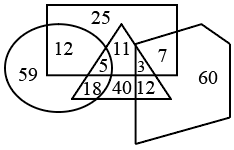Question 9

# The data of a company’s employees is shown by the given Venn diagram. The circle represents ‘engineers’, the triangle represents  computer experts’, the rectangle represents ‘MBAs’ and the pentagon represents ‘females’. The numbers given in the diagram represent the number of persons of that particular category.How many of the employees are MBAs, and how many male MBAs are computer experts but NOT engineers, respectively?

Solution

Given that  The circle represents ‘engineers’, the triangle represents computer experts’, the rectangle represents ‘MBAs’ and the pentagon represents ‘females’then the employees are MBAs, and how many male MBAs are computer experts but NOT engineers

MBA's = 25 + 12+ 5+ 11+3+7 = 63 (from the rectangle represent the MBA)

and male MBAs are computer experts but NOT engineers = 11 (From the above representation )

therefore the employees are MBAs, and how many male MBAs are computer experts but NOT engineers= 63, 11

Hence Option (B) 63,11 Ans